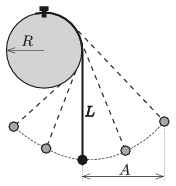Mathematical and Physical Journal
for High Schools
Issued by the MATFUND Foundation
 Already signed up? New to KöMaL?

#Problem M. 382. (December 2018)

M. 382. One end of a thin, flexible and not stretchable thread is attached to the topmost point on the rim of a cylinder of radius $\displaystyle R$. The cylinder is fixed and it has a horizontal axis. A small object is attached to the other end of the thread. In the equilibrium position the vertical part of the thread has a length of $\displaystyle L=3R$. The object is displaced, as shown in the figure, and then it is released. The period of the motion – for a relatively large initial displacement – of the object depends on the ''amplitude'' $\displaystyle A$. Measure for several different values of $\displaystyle A$ by what percent the period of this pendulum $\displaystyle T(A)$ differs from the period $\displaystyle T_0=2\pi\sqrt{{L}/{g}}$ of a simple pendulum of length $\displaystyle L$?(6 pont)

Deadline expired on January 10, 2019.

### Statistics:

 11 students sent a solution. 6 points: Kondákor Márk, Kozák 023 Áron, Olosz Adél, Pácsonyi Péter. 5 points: Fülöp Sámuel Sihombing, Morvai Orsolya, Varga 235 Áron. 4 points: 2 students. 3 points: 2 students.

Problems in Physics of KöMaL, December 2018